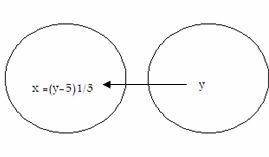Email us to get an instant 20% discount on highly effective K-12 Math & English kwizNET Programs!

Online Quiz (WorksheetABCD)

Questions Per Quiz = 2 4 6 8 10

High School Mathematics - 24.5 Onto and Into Functions

 The mapping f: A -> B is called an onto function if the set B is entirely used up, i.e if every element of B is the image of atleast one element of A. For every b € B there exists atleast one element a € Asuch that f(a) = bIf function f: A -> B is not onto, that is some of the elements of B remain unmated, then f is called an into function. Remarks: An onto function is also called surjective function or a surjection. A one-one function may be both onto and into. If A and B are finite sets and f: A->B is surjective, then n(B) <= n(A). If n(A) = m, n(B) = m, then the possible number of possible surjective mappings from A to B is m!. : Let A = {-2,-2, 3,3}, B = {4,9} and f: A ->B be a function defined by f(x) = x2, then f is onto because f(-2) = 4, f(-3) = 9, f(3) = 9 i.e f(A) = {4,9} = B. If A and B are finite sets and f: A ->B is surjective, then n(B) <= n(A). If n(A) = m and n(B) - m, then the possible number of possible surjective mappings frm A to B is m!. Example: Let A = {-2, 2, -3, 3}, B = {4,9} and f: A -> B be a function defined by f(x) = x2, then f is onto, because f(-2) and f(2) = 4 and f(-3) and f(3) = 9,i.e f(A) = {4,9} = BA function f: N ->N defined by f(x) = 7x is not an onto function, because f(N) = {7,14,21,..} is not equal to N(co-domain). Method to find surjectivity of s function f: R -> R defined by f(x) = x3 + 5 for all x € R. Choose any arbitrary element y in B. Put f(x) = y Solve the equation f(x) = y for x and obtain x in terms of y. Let x = g(y). If for all values of y € B, the values of x obtained from x = g(y) are in A, then f is onto. If there are some y € B for which x, given by x = g(y), is not in A, then f is not onto. Solution: Let there be an arbitrary element in R. Then f(x) = y = x3 + 5 x = (y -5)1/3. Now for all y € R, (y-5)1/3 is a real number. So for all y € R (co-domain), there exists x = (y - 5)1/3 in R (domain) such that f(x) = x3 + 5Here f: R -> R is an onto function. Directions: Answer the following.
 Q 1: {(x, y) : y = 5x - 6 for all x belongs to W}. If this is a function, what type of function is this? Not a functionOntoOne-one Q 2: {(x, y) : y = 5x - 6 for all x belongs to W}yesno Question 3: This question is available to subscribers only! Question 4: This question is available to subscribers only!